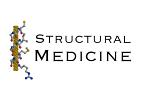# Protein Crystallography CourseCourse Homepage   Basic: 1 2 3 4 5 6   Advanced: 7 8 9 10 11 12 13 14 15 16 17 18 19 20 21 22

# Concepts

One way to solve the phase problem is to possess an atomic model, from which estimates of the phases can be computed. Such a model can be obtained when you know the structure of a related protein, or even the same protein from a different crystal form. But to build up an atomic model of the new crystal form, it is necessary to work out how the model should be oriented and position in the new cell. Molecular replacement is the technique used to solve that problem.

Molecular replacement can be used to solve a structure when you have a good model for a reasonably large fraction of the structure in the crystal. As the database of solved structures gets larger and larger, this method will be useful for a larger fraction of new structures. Of course, it is also useful for binding studies or studies of complexes formed from previously-determined proteins.

The level of resemblance of two protein structures correlates well with the level of sequence identity, which means that you can get a good idea of whether or not molecular replacement will succeed before even trying it. As a rule of thumb, molecular replacement with modern programs will probably be fairly straightforward if the model is fairly complete and shares at least 30% sequence identity with the unknown structure. It becomes progressively more difficult as the model becomes less complete or shares less sequence identity.

## Patterson-based vs. likelihood-based

Traditionally, molecular replacement has been based on the properties of the Patterson function. Patterson-based methods are still used in programs such as Molrep and AMoRe, so it is worth understanding how they work. Likelihood-based methods are now playing an increasing role, particularly for difficult molecular replacement problems, because likelihood provides a more sensitive target. However, it is easier to gain an intuitive understanding of the Patterson-based methods so we will begin by exploring those.

The Patterson function is important in crystallography because it can be computed without phase information. We can also compute a Patterson from a trial atomic model and compare it to the observed Patterson. When the model is oriented correctly and placed in the correct position in the unit cell, the two Pattersons should be similar. In this simplistic approach (which may in fact be useful under special circumstances!), the molecular replacement problem has six dimensions (three parameters to specify orientation and three to specify position), which makes for a very large problem. Fortunately, the Patterson map can be divided into parts that are sensitive to only subsets of these parameters. The usual strategy is to look at these parts separately, reducing the size of the problem. Similarly, as we will see, it is possible to define likelihood targets that are sensitive only to some of the orientation/translation parameters.

## Divide and conquer

Most molecular replacement programs use a divide-and-conquer strategy, by determining first the orientation (three parameters) and then the translation (also three parameters).

Remember that the Patterson map is a vector map, with peaks at the positions of vectors between atoms in the unit cell. This is illustrated in the following two figures. The first one shows four unit cells of a small crystal.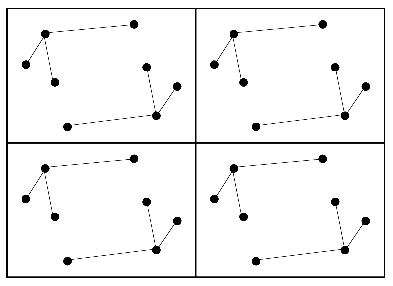The vectors between these atoms give rise to the following Patterson map.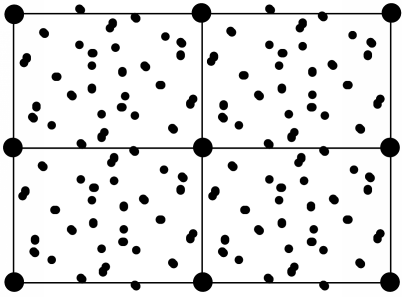Even though the vectors are unresolved for a structure the size of a protein, the way that they accumulate can provide a signature for a protein structure. The vectors in the Patterson map can be divided into two categories. Intramolecular vectors (from one atom in the molecule to another atom in the same molecule) depend only on the orientation of the molecule, and not on its position in the cell, so these can be exploited in the rotation function. Intermolecular vectors depend both on the orientation of the molecule and on its position so, once the orientation is known, these can be exploited in the translation function.

Actually, there is another way to think of this, which is to consider that some parts of the Patterson reflect subsets of the space group symmetry. The intramolecular vectors come from the P1 symmetry (just the identity). A full 3D translation function considers the rest of the symmetry, but it is also possible to consider intermediate subsets of the symmetry. For instance, in the space group P222, three subsets of the symmetry have space group P2, with only a single two-fold axis along x, y or z. These subsets of the symmetry will generate subsets of the intermolecular vectors that are sensitive to only the components of the translation perpendicular to the particular symmetry axis. This should be clearer when we look at translation functions, which can be 2D as well as 3D.

# Rotation function

The rotation function, as just mentioned, exploits the fact that the intramolecular vectors depend only on the orientation of the molecule, not on its position in the unit cell. To see why this is so, you should consider what happens when you translate a molecule in the cell. All of the atoms within that molecule are shifted by the same amount, but the vectors between them remain the same.

The use of intramolecular vectors to orient a molecule is illustrated schematically in the following figures. The first figure shows the molecule in the crystal, but in a random orientation (left), together with its set of intramolecular vectors (right).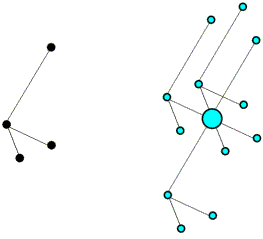Next is shown the Patterson map from above, but to make it easier to see what is going on the vectors are colour-coded. The intramolecular vectors are light blue.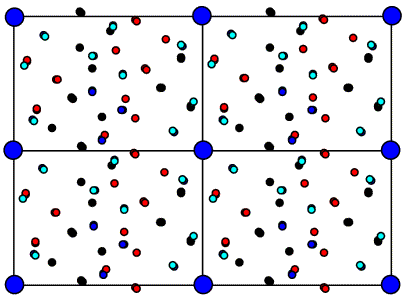Now if we rotate the molecule, we rotate its intramolecular vectors in the same way, and we can see that the oriented vector set below matches the light blue peaks in the Patterson above.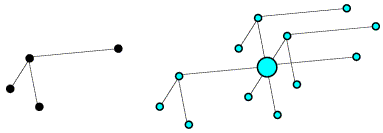On average, the intramolecular vectors will be shorter than the intermolecular vectors, so the rotation function can be computed using only the part of the Patterson map near the origin, which should reduce noise arising from the intermolecular vectors.

## Classic rotation function

Before defining the rotation function mathematically, we have to consider how to describe orientations. Describing an orientation requires three parameters, but there are different choices of how to describe it, depending on what you are doing and how you want to visualise things.

One way to define an orientation is to define a rotation axis and an angle of rotation about that axis. It takes two parameters to define an axis, which you can think of as a vector from the centre of a sphere to a point on the surface of the sphere. One common convention is shown below. The rotation axis starts off parallel to z and is rotated around the y axis by an angle ψ. Then it is rotated around the z axis by an angle φ. These specify a point on the surface of a unit sphere, analogous to defining a point on the earth's surface by latitude and longitude. Finally, the object being oriented is rotated by an angle κ around the rotation axis.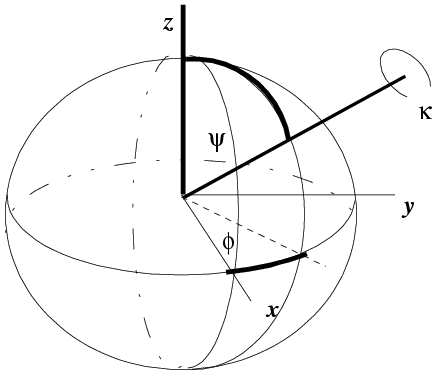(illustration courtesy DJ Derbyshire)

The κ/φ/ψ description is particularly useful if you are looking for rotations with a particular rotation angle (κ). For example, 2-fold rotations will have κ=180°, while 6-fold rotations will have κ=60°.

Another convention, more common in molecular replacement, is to use Euler angles. These can be defined in various ways, but the one you will come across in molecular replacement programs such as AMoRe is the zyz convention. In this convention, the coordinate system is rotated by an angle α around the original z axis, then by an angle β around the new y axis, and then by an angle γ around the final z axis.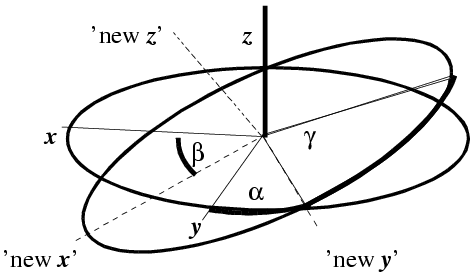(illustration courtesy DJ Derbyshire)

The Euler angle description is particularly useful for the mathematics of the fast rotation function, discussed briefly below.

With that as background, the rotation function is commonly defined as a product function. The model Patterson is rotated and superimposed on the observed Patterson. The product of the two Pattersons at all points in a spherical shell is summed, and that is the rotation function score. In the equation shown below, the rotation is expressed in terms of κ/φ/ψ.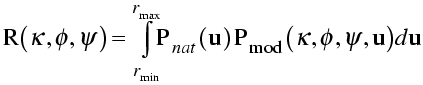In the integration over a spherical shell, a region near the origin is typically omitted to leave out the large origin peak of the Patterson, which would add a large constant term. The sphere is limited in radius because the intramolecular vectors are more concentrated near the origin. Also, the maximum radius should not be long enough to reach another lattice point (and its associated origin peak).

## Fast rotation function

Originally, the rotation function was computed by directly comparing two Pattersons or lists of peaks in those Pattersons. Most programs now use a fast rotation function, based on an algorithm originally developed by Tony Crowther. He realised that the rotation function could be computed quickly with Fourier transforms, if the Pattersons were expressed in terms of spherical harmonics. We won't go into details, but the maths are similar in spirit to the convolution theorem.

The rotation function in AMoRe is based on the same theory, but Jorge Navaza realised that the original implementation of the fast rotation function suffered from numerical instabilities in computation. The improved numerical analysis can increase the signal-to-noise significantly.

## Direct rotation function

In the rotation functions considered so far, the model Patterson is computed for a different unit cell than the observed Patterson, chosen to minimise interference from molecules related by unit cell translations. However, this introduces approximations, and vectors from the molecule to its neighbours in neighbouring unit cells are not considered. In the direct rotation function, the molecule is placed in the unit cell of the unknown structure, and the Patterson for the oriented molecule is compared with the entire Patterson of the unknown structure.

# Translation function

Traditional translation functions compare sets of intermolecular vectors (which depend on the position of the molecule in the unit cell) with the observed Patterson. How this works can be seen in the schematic figures below.

In the figure on the left, the correctly-oriented model is put in an arbitrary position in the unit cell. Application of the crystallographic two-fold generates a second copy of the molecule. When this molecule is moved to its correct position (magenta arrow in the figure on the right), the copy moves in the opposite direction relative to the two-fold axis.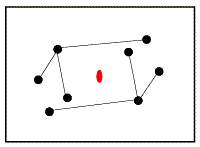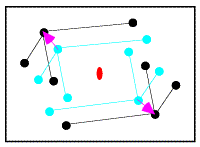From the model on the left above, it is possible to compute a set of intermolecular vectors from atoms in the original molecule (upper left) to the one generated by the two-fold symmetry (lower right). These intermolecular vectors are shown in the figure below.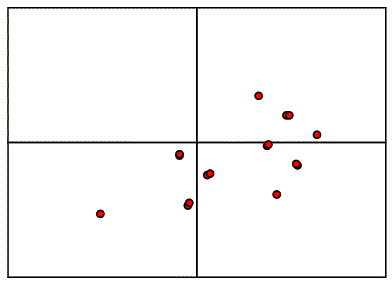If we were to move the model relative to the two-fold axis, as shown above, all the vectors would shift by double that amount, because the symmetry-related copy shifts in the opposite direction. So when we compare the intermolecular vectors above to the corresponding vectors in the Patterson map below (colour-coded for convenience), we have to divide the relative shift by two to work out where to move the model.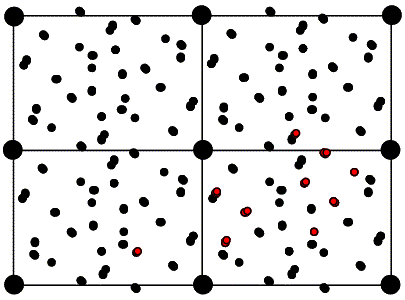## Targets: product function vs. Patterson correlation

As with the rotation function, the traditional definition for the Patterson-based translation function was a product function. First we need a mathematical definition of the subset of the calculated Patterson corresponding to the intermolecular vectors. What we will want is the vectors from the symmetry-related copy (ρ2) to the original copy, (ρ1). Just as the Patterson map is an autocorrelation function (i.e. a correlation function of the density with itself), the intermolecular vector set is a correlation function between the density for one molecule and the density for the other molecule, as shown in the following figure and equation. Of course, the correlation function could be computed with a single Fourier transform, using the correlation theorem discussed previously. But we will just save the Fourier coefficients we would use for this for a moment.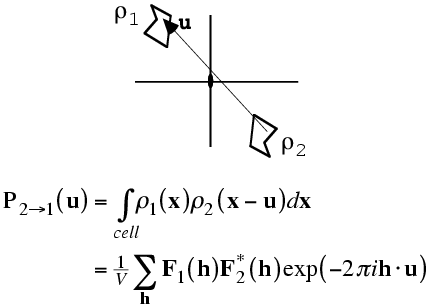The required translation, relative to the two-fold axis, can be deduced by translating the intermolecular vectors over the observed Patterson map and computing a product function. When the correct translation is chosen, there should be a large peak because the vector sets will coincide.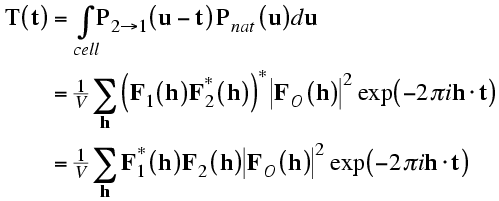The correlation theorem means that this translation function can be computed with just a single Fourier transform.

Of course, for the reasons discussed above, the translation that superimposes the calculated intermolecular vectors on the observed intermolecular vectors has to be divided by two, in order to determine the translation to apply to the model. As well, note that this translation function only tells us the component of the translation perpendicular to the rotation axis. For higher symmetry space groups, such as P222, we can compute such translation functions for each symmetry axis, which gives us all the components of the translation vector.

It is easier to interpret a function that gives the translation vector directly, and signal-to-noise can be improved by considering all the symmetry operations simultaneously. One popular way to do this is to compute the correlation coefficient between the observed and calculated amplitudes squared, as a function of model translation. The correct translation should give a peak in this map.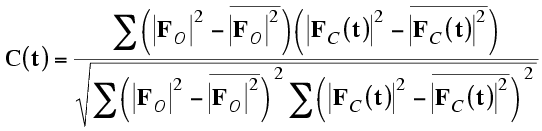It turns out, because of Parseval's theorem, that this correlation coefficient is equivalent to the correlation coefficient between the two origin-removed Patterson maps. For that reason, this is often called the Patterson correlation (PC) score.

As shown originally with the program BRUTE, which carries out a brute-force rotation/translation search by computing this correlation score, PC searches can be carried out using a subset of the space group symmetry. If only the identity is given (P1), this gives a direct rotation function. In this case, the calculated Patterson possesses the intramolecular vectors but lacks the intermolecular vectors. Nonetheless, the two Pattersons are positively correlated at the correct orientation. Alternatively, just the symmetry operations corresponding to one axis can be given and then the score is sensitive to orientation and the two components of translation perpendicular to the axis.

PC searches are now generally carried out more conveniently with X-PLOR and CNS. In addition, AMoRe gives PC scores as the primary way to judge the quality of trial molecular replacement solutions.

## Search volumes

In conducting a translation search, you might think that it is necessary to search over the asymmetric unit. In fact, this is not true. The origin of a crystal is, to a certain extent, arbitrary. In P1, for instance, any point can be an origin, so it is not necessary to carry out a translation search at all. In higher symmetry space groups, the mathematical form of the symmetry operations restricts the possible origins, but there are still different valid choices. So all that needs to be searched is the unique volume relative to any allowed choice of origin. For an orthorhombic space group, the two-fold axes (rotational or screw) repeat every half unit cell in all three directions, so there are eight possible choices of origin. In contrast, the asymmetric unit for (say) P222 occupies a quarter of the unit cell.

This unique volume relative to a possible choice of origin is called the Cheshire cell. It was probably named by a fan of Lewis Carroll, because the Cheshire cell is what is left when you take away the contents of the unit cell and leave only the symmetry operators (read "smile").

Mind you, you are only limited to the Cheshire cell in searching for the first molecule, when there is more than one molecule in the asymmetric unit. Placing the first molecule fixes the origin, so subsequent searches for additional molecules have to cover the whole unit cell (or, to be precise, the unique volume relative to a lattice point; the necessary search volume is reduced in a centered lattice).

Determining the Cheshire cell used to be the greatest intellectual challenge in running BRUTE. Modern programs work out the necessary search volume for you.

# Searches in higher dimensions

When there is more than one molecule in the asymmetric unit, the size of the problem grows dramatically. Six degrees of freedom are required to specify each molecule. Fortunately, it is still usually possible to solve the rotation and translation separately for each molecule, starting preferably with the best model (largest fraction and/or highest sequence identity).

If there is more than one copy of the same molecule, a self-rotation funcion can be computed, comparing the Patterson with a rotated copy of itself. Peaks in this function indicate rotations that will put one molecule into the orientation taken by another molecule. The existence of a strong self-rotation peak puts strong constraints on the orientations of pairs of molecules.

There is a (very common) special case in which no self-rotation peak is seen. Often, when there is more than one copy of a molecule in the asymmetric unit, the non-crystallographic symmetry axis runs nearly parallel to the crystallographic symmetry axis. This is shown schematically in the figure on the left below, where it can be seen that the combination of 2-fold crystallographic and non-crystallographic symmetry generates translational pseudo-symmetry. In other words, the two dimers in the figure are related by a translation, even though pairs of monomers related by that translation are not symmetry equivalents. There will be pairs of atoms related by the vector from one dimer axis to the other; their contributions to the native Patterson function will all add up to give a very large peak, as shown on the right.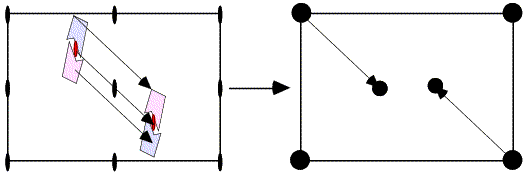When a large peak (a substantial fraction of the origin peak) is observed in a general position in a native Patterson map, this is a strong indication of translational pseudo-symmetry, which reduces the unknowns considerably.

These tricks do not always work and, of course, some crystals contain complexes of different molecules. It is sometimes very difficult, in such a case, to determine if the answer is correct until more than one molecule has been placed. One approach is to attempt to place more than one molecule simultaneously using Monte Carlo (with the program Queen of Spades) or genetic algorithms (with EPMR). Another is to conduct a search tree, in which all plausible solutions for one molecule are used as a starting point for the search for subsequent molecules, as done in Phaser.

# Exploiting phase information

So far, we have assumed that we have only native diffraction data and a molecular replacement model. Of course, we can well find ourselves in a situation where we have experimental phases as well as a model. Phase information can, in fact, be exploited to help to solve the molecular replacement problem. This is particularly useful when we have a molecular replacement model that is too poor to find a solution and phases that are too poor to provide an interpretable map. The two together may be enough to succeed.

## Domain rotation function

As with standard molecular replacement, phased molecular replacement can separate the rotation and translation problems. The phased equivalent of the rotation function is called the domain rotation function, invented by Peter Colman. The figure below illustrates a domain rotation function for discovering the rotation relating two molecules in an experimental density map, which is useful (as we will see in a later lecture) for averaging calculations. Basically, the map need only be good enough to make a rough guess of where the molecules are located. Then spheres of density can be cut out, placed in an empty unit cell, and transformed to get structure factors. These structure factors are then used to compute a normal rotation function. Of course, one set of structure factors can come from a model instead of a map.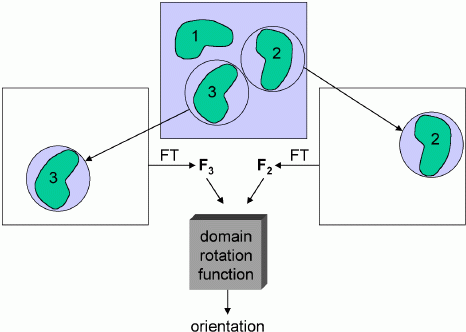You might think that poor phase information would give poor amplitudes for a rotation function. But it seems that, by cutting out the density for a single molecule, the phase information allows us to simplify the Patterson function tremendously, eliminating all the intermolecular vectors and other intramolecular vectors. The reduction in noise more than compensates for the phase errors.

## Phased translation function

Once the orientation is known, the density (or model) can be oriented and used to compute structure factors. Then a correlation function can be computed to determine the vector that translates the oriented density (or model) on top of the similarly-oriented density in the map.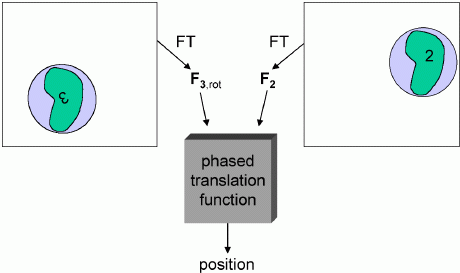In the schematic below, the left side shows an oriented but arbitrarily positioned density. If it were translated by the vector t, it would superimpose well on the experimental density for the corresponding molecule on the right side. The density superposition can be judged by a product function, computed using the correlation theorem as a single Fourier transform.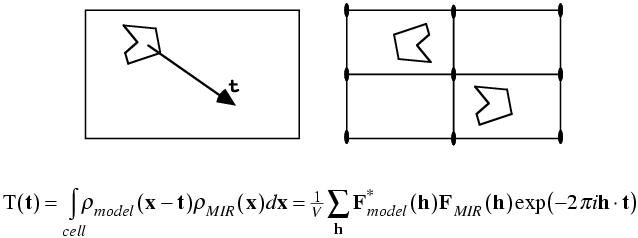# Likelihood-based molecular replacement

We have seen that likelihood is a much better target for judging the quality of an atomic model than the traditional targets. It turns out that likelihood is also a better target for molecular replacement.

One advantage to likelihood, as in the case of structure refinement, is that arbitrary parameters become much less arbitrary. For instance, when you are computing a traditional rotation function, you have to choose the resolution range of the data, whether or not to use normalised or sharpened data (and if so, sharpened by how much), integration radii, and whether or not to include side chains. There are rules of thumb for these parameters (use lower resolution data with less sharpening for a poorer molecular replacement model), but they are a matter of judgement. With likelihood, we know (as we will see below) how model error will affect our ability to predict the structure factors. If we can make a good guess of the error levels in the model, the likelihood target will pay appropriate attention to data at different resolution values. (And we can make a reasonable guess of error levels, because rms errors correlate well with overall sequence identity.) So we can, in principle, use all the data in the calculations, knowing that the likelihood target will effectively ignore data from too high resolution. In principle, we could also predict different levels of coordinate error for atoms from main-chain and side-chains, instead of making an arbitrary choice to eliminate some atoms.

## Principle of maximum likelihood

The basic principle of maximum likelihood can be summarised as follows: the best model is the one that is most consistent with the data, and consistency with the data can be measured by the probability that the data would be measured given the model that is being tested. This is explored in more detail in the lecture on likelihood and refinement.

Likelihood is often used to test hypotheses, and one way to consider molecular replacement is that it is testing a series of hypotheses. For instance, in a rotation search you are testing, for each set of orientation angles, the hypothesis that a molecule in the target unit cell resembles the search model in the orientation specified by those orientation angles. To test the hypothesis, we assume that the model is in the correct orientation and then see whether there would be a high probability that the data could be observed, given the model in that orientation.

To compute such a probability, you need to understand the sources of error in using the model to predict the data. We've looked at this in the context of refinement, where the effect of errors in the model can be described using a parameter σA. The refinement likelihood target is appropriate for a translation search using a model in a known orientation, because each possible translation generates an atomic model with, for the correct translation, all the atoms in about the right place. However, if we consider a rotation search, if we specify the orientation of a molecule we still do not know its position in the unit cell, and this uncertainty introduces new errors into the prediction of the data.

## Likelihood-based rotation function

The rotation function tests whether an orientation for the search model is consistent with the data. In computing the probability used for the likelihood target, we assume that the model is in the correct orientation but that nothing is known about its position in the unit cell. If you look at the structure factor equation and consider a set of molecules making up a molecule, if you change the position of the molecule you will be adding the same translation vector to all the atoms, which will introduce the same phase shift to the contributions of all those atoms to the overall structure factor. So translating a molecule only changes the phase of its contribution to a structure factor, but does not change the amplitude. On the other hand, rotating the molecule will change both its amplitude and its phase. Now, if a molecule is translated in a crystal, then the symmetry-related copies will be translated in symmetry-related directions, which are usually different, so there will be different phase shifts applied to the different symmetry-related molecules. That is why the overall structure factor is sensitive to the translation of the molecule.

The animation below illustrates what happens as we translate a molecule in space group P6. There are six contributions from symmetry-related molecules and, as we translate the first copy, the phases of all six contributions change in different ways. In terms of probabilities, the way we can think of this is that, when you know the amplitudes of the contributions from the six molecules but not their relative phases, the total structure factor is obtained by a random walk with steps of known length but unknown direction. (For the moment we will ignore the effects of errors and incompleteness in the model.)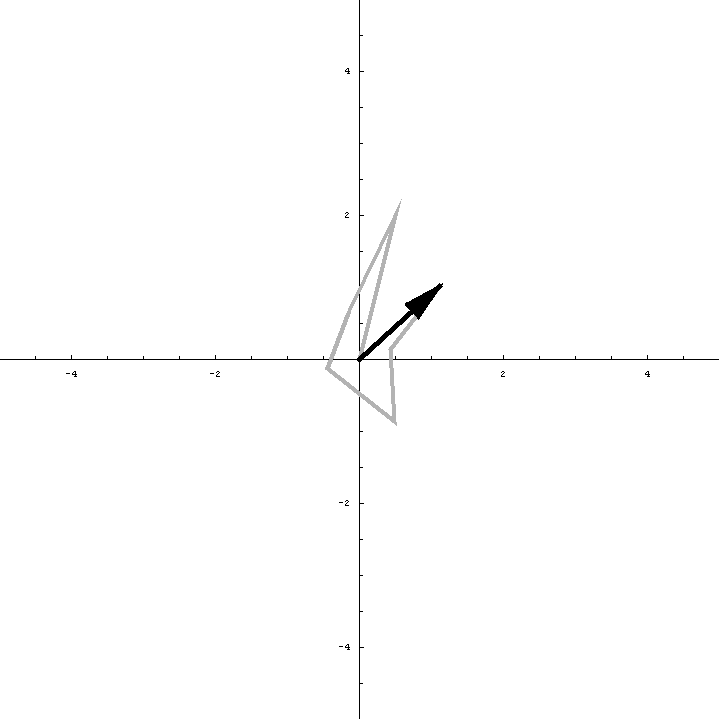As we saw in the discussion of the Wilson distribution in the lecture on crystallographic statistics, the sum of the steps in a random walk (i.e. where the walk ends) has a distribution that can be approximated as a 2D Gaussian, and the variance of this Gaussian is equal to the sums of the squares of the lengths of the steps. So the probability distribution for a rotation search can also be approximated as a 2D Gaussian, with the variance being given by the sum of the squares of the contributions of each of the individual molecules to the total structure factor. As we rotate the model (and apply symmetry-related rotations to the symmetry-related copies), we change the amplitude of the contributions of these molecules to the total structure factor, and this changes the probability distribution. As for the Wilson distribution, to get the probability distribution for the amplitude, we have to integrate over all possible values of the phase.

Remember that the Wilson distribution was based on the assumption that the conditions of the Central Limit Theorem apply. One of these assumptions, that there is a large number of contributions to the sum, is much weaker in the case of the rotation likelihood function, but this does not seem to be a problem in practice. This is partly because the random walk of symmetry-related structure factors is far from the only source of uncertainty, particularly for difficult molecular replacement problems.

There are two other significant sources of uncertainty. First, the molecular replacement model is not exactly the same as the target in the unknown crystal. Most of the time even the sequence will be different, so not all the atoms will be in one-to-one correspondence. Even the atoms that have a match will be in different places and will have a different distribution of B-factors. As we saw when we were looking at distributions of pairs of related structure factors, the effect of model errors is that the center of the probability distribution for the true structure given the model is obtained by multiplying the model structure factor by D (a factor generally less than one), and the distribution is a 2D Gaussian, which becomes broader as the model becomes worse. So to take account of model errors in the rotation likelihood target, we have to multiply the contributions of the symmetry-related models by D and add the uncertainty in their true values to the overall 2D Gaussian distribution for the sum.

The second source of uncertainty is incompleteness in the model. As we saw for the Sim distribution, atoms with unknown position also add to the variance of the 2D Gaussian.

It turns out that we can do a bit better than the Wilson distribution as a rotation likelihood function. All we are worried about is the amplitude of the total structure factor, not its phase, so all that matters is the relative phases of the contributions from the symmetry-related molecules, not their absolute phases. This means that we can fix one of the phases arbitrarily and consider what happens as the phases of the other molecules vary. In other words, we look at a random walk that starts from the end of one of the contributions to the structure factor. This reduces the variance of the 2D Gaussian and thereby sharpens the likelihood function. If we pick the molecule with the largest contribution to the total structure factor, we get the maximum reduction in the variance and thus the most sensitive rotation likelihood target. This is illustrated in the animation below, where the single largest contribution has been fixed and the other contributions rotate around it. The blue shading indicates the 2D Gaussian distribution that arises from the random walk plus the effects of model error and incompleteness.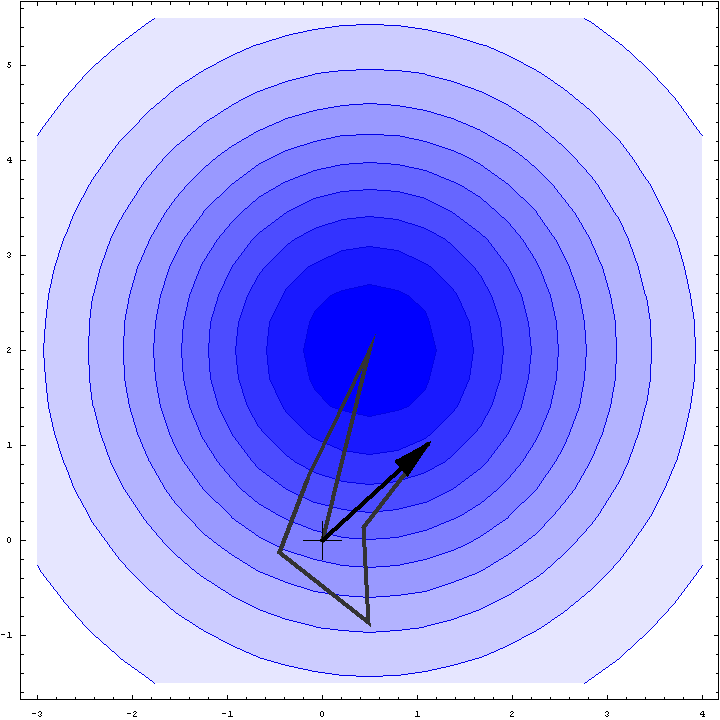This rotation likelihood target is a type of direct rotation search, because it is computed in the unit cell of the target and considers the contributions from all symmetry-related copies. As such, it tends to be very computationally intensive to evaluate. The program BEAST, which was developed to carry out brute-force likelihood-based molecular replacement searches, was effective on some difficult problems but was too slow for routine use.

To speed up such searches, fast approximations to the brute-force likelihood targets were implemented in Phaser. Briefly, the fast rotation search is obtained by taking the first-order term from a Taylor series approximation to the brute-force likelihood target, then expanding this function in terms of spherical harmonics, just as is done for the fast rotation function based on Patterson methods. Effectively, when searching for the first molecule, the likelihood-based fast rotation function is equivalent to a Patterson-based search, in which the origin is removed and the terms are downweighted according to their expected error.

The rotation likelihood target also allows one to exploit information from knowing part of the structure, for example when the structure of a complex is solved. The fixed (rotated and translated) molecule just adds another contribution to the total structure factor, which can be treated in the same way as the contributions from symmetry-related components. This can increase the signal for a second molecule significantly.

## Likelihood-based translation function

As noted above, the likelihood-based translation function is the same as the likelihood refinement target, because each point in a translation search predicts the total structure factor, even if there is a fixed known component. In contrast to the rotation case, uncertainty comes only from errors and incompleteness of the model.

It is possible to carry out a translation search exploiting the knowledge of the orientation, but not the position, of another component in the structure; in this case, the same likelihood target as for the rotation search is used, as the uncertainty in the relative phases of the contributions from the fixed component give rise to a random walk. This option was implemented in BEAST and in the initial versions of Phaser, but in practice it is a better strategy to carry out a translation search for the first molecule before attempting to find the second molecule.

As for the rotation likelihood function, the translation likelihood function is computationally intensive. But, in a similar fashion, it is possible to compute the first-order term of the Taylor series approximation quickly, using an FFT-based calculation.

## Calibrating the likelihood targets

To compute the likelihood targets, it is necessary to have values for some parameters, i.e. the factor D (the fraction of the calculated structure factor that is correlated to the true structure factor) and the variance of the distribution (the expected mean-square error of the D-weighted calculated structure factor). Both of these parameter can be calculated by knowing the value of σA as a function of resolution. In refinement, σA is estimated from the cross-validation data, by comparing the observed and calculated structure factor amplitudes, but in molecular replacement the calculated structure factor amplitudes are not known before the structure is solved.

To get around this problem, we can use a good initial guess for the σA curve. If we assume that errors in the calculated structure factors come from coordinate errors with a known Gaussian distribution and from incompleteness of the model, then there is a relationship that will give the σA curve as a function of resolution. Generally we have a good idea of the content of the asymmetric unit (from the molecular weights of the components, their expected stoichiometry, and the knowledge that most protein crystals have about 50% solvent), so we know how complete the model is. The RMS error of the model can be estimated from a curve derived by Cyrus Chothia and Arthur Lesk, who observed that the RMS deviation between the main-chain atoms of related proteins is highly correlated to their sequence identity.

## Automation in Phaser

Phaser has a number of automation features to make it easier to solve difficult molecular replacement problems. Problems with several molecules in the asymmetric unit tend to be difficult, even if the models are good, because the signal from each individual molecule is weak. Phaser deals with these by doing the book-keeping required for a highly branched search. At each step, Phaser keeps a list of potential partial solutions. In the next step, each of these potential partial solutions is tested in turn. The correct partial solution will tend to lead to a solution with a higher signal in a later stage, so that an ambiguous potential solution may become an unambiguous solution.

In difficult cases, there may be a choice of several possible models, which may be distant relatives of each other (and of the target) or may have different relative orientations of domains. Phaser allows these alternative models to be tested in turn. Alternatively, it is possible to superimpose alternative models on each other and then use a statistically-weighted ensemble average as the model. (However, this strategy does not make sense if the alternative models superimpose poorly on each other.)

Finally, there may be some ambiguity about choice of space group, either because the zones of diffraction data where systematic absences would distinguish among the possibilities were not collected, or because the space group is part of an enantiomorphic pair. All possible choices of space group can be tested in a single run.

## References

The Phaser web page gives more detail on the methods and documentation for running the program. The underlying theory and algorithms are described in papers available as PDF files.